### Home > CCG > Chapter 11 > Lesson 11.2.3 > Problem11-117

11-117.

Solve for the variables in each of the diagrams below. Assume that point $C$ is the center of the circle in part (b).

1.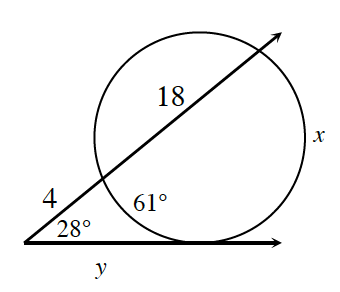$\tan^2=\text{ext. secant segment}$
(ext. sec. seg. + int. sec. seg.)
$y^2 = 4(4 + 18)$

and

$28=\frac{x-61}{2}$

1.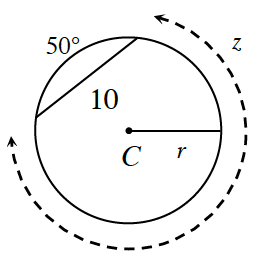Draw a perpendicular bisector on the chord to the center of the circle, and then create two triangles.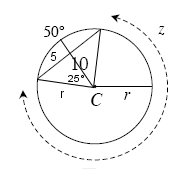$\sin25º = \frac{5}{r}$

$r ≈ 11.83$

1.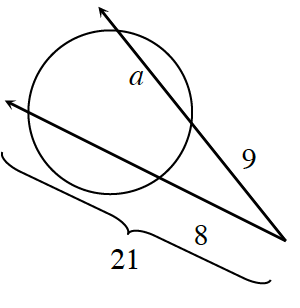Draw two intersecting chords, which will create two congruent triangles.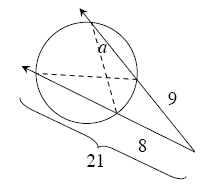$\frac{a+9}{8}=\frac{21}{9}$